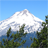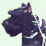# Updating fields using python in ArcGIS 10

2633
2
10-31-2011 08:26 AMNew Contributor
I have 10 fields that have a floating value between 0 and 1.  These fields have a meaningless name, so they've been assigned aliases.  I also have three fields called 'First', 'Second', and 'Third' that are empty.  Using python, I want to find the first, second, and third highest of the 10 values for each record and assign the field alias to the First, Second, and Third fields respectively.

For instance, in a given record, if the highest value of the 10 floating numbers was from the field with the alias 'NF', then the field 'First' would get the value 'NF'.  I've been at this for a few hours and I can't seem to make it work.  Any help would be much appreciated.
Tags (3)
2 Repliesby
New Contributor
You can put your 10 fields into a list and sort it.
Then the first, second and third value of this list can be written to your 3 fields.New Contributor
Thanks, Ben.  That's what I ended up doing.  Here's the code in case anyone's interested.  I'm sure someone else out there could do it in less lines of code, but it works nonetheless.

`import osfrom operator import itemgetterimport arcpyinspace = "C:/Data/export.gdb"shapefile = inspace + os.sep + "classification_2011_1028"fields = arcpy.ListFields(shapefile, "M*") # The "nonsense" name of the 10 fields all began with 'M'newfields = ["First", "Second", "Third"] # The empty fields where the top three numbers would gouCursor = arcpy.UpdateCursor(shapefile)for row in uCursor:    fuzzy = []    topthree = []    x = 1    for field in fields:        name = field.aliasName         value = row.getValue(field.name)        fuzzy.append([name,value])    fuzzy.sort(key=itemgetter(1),reverse=True)    for f in fuzzy:        if x < 4:            topthree.append(f)            x+=1    for rank, value in zip(newfields,topthree):        row.setValue(rank,value)    uCursor.updateRow(row)    `